ISEE Lower Level Quantitative : Plane Geometry

Example Questions

What area of the rectangle is filled in?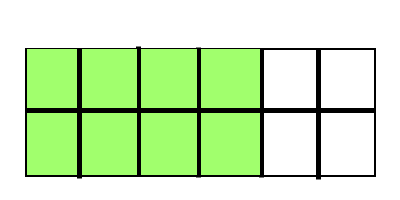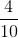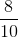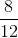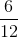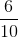Explanation:

Area is the amount of space within the perimeter of a two-dimensional shape. The rectangle is split intopieces andof those pieces are filled in.of the area of this rectangle is filled in.

What area of the rectangle is filled in?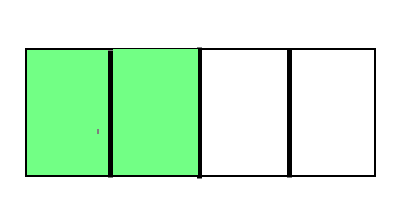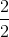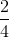Explanation:

Area is the amount of space within the perimeter of a two-dimensional shape. The rectangle is split intopieces andof those pieces are filled in.of the area of this rectangle is filled in.

What area of the rectangle is filled in?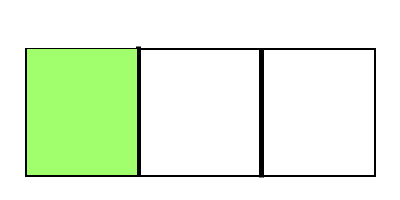Explanation:

Area is the amount of space within the perimeter of a two-dimensional shape. The rectangle is split intopieces andof those pieces is filled in.of the area of this rectangle is filled in.

What area of the rectangle is filled in?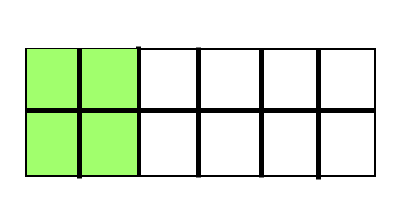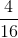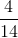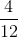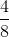Explanation:

Area is the amount of space within the perimeter of a two-dimensional shape. The rectangle is split intopieces andof those pieces are filled in.of the area of this rectangle is filled in.

What area of the rectangle is filled in?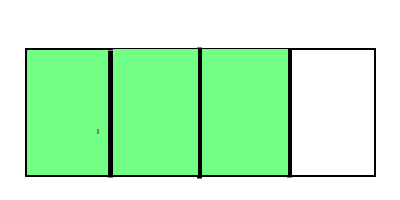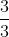Explanation:

Area is the amount of space within the perimeter of a two-dimensional shape. The rectangle is split intopieces andof those pieces are filled in.of the area of this rectangle is filled in.

What area of the rectangle is filled in?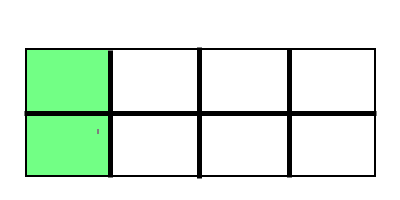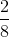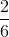Explanation:

Area is the amount of space within the perimeter of a two-dimensional shape. The rectangle is split intopieces andof those pieces are filled in.of the area of this rectangle is filled in.

What area of the rectangle is filled in?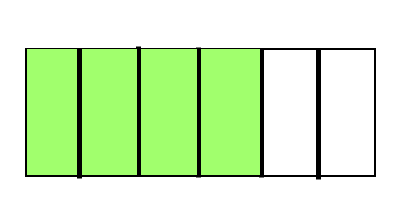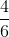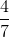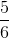Explanation:

Area is the amount of space within the perimeter of a two-dimensional shape. The rectangle is split intopieces andof those pieces are filled in.of the area of this rectangle is filled in.

What area of the rectangle is filled in?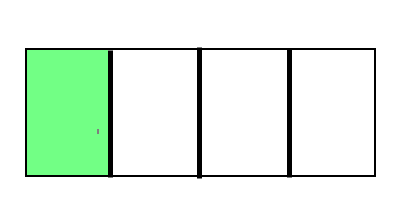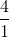Explanation:

Area is the amount of space within the perimeter of a two-dimensional shape. The rectangle is split intopieces andof those pieces is filled in.of the area of this rectangle is filled in.

Example Question #1 : Geometric Measurement: Understanding Concepts Of Area And Relating Area To Multiplication And To Addition

How many square units make up the area of the shape below?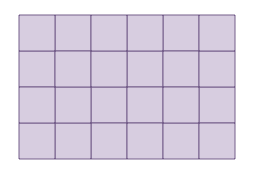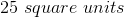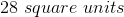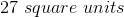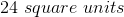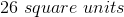Explanation:

The shape is made up of unit squares. We can count the number of squares within the shape to find the area.

There aresquares within the shape.

Example Question #2 : Geometric Measurement: Understanding Concepts Of Area And Relating Area To Multiplication And To Addition

How many square units make up the area of the shape below?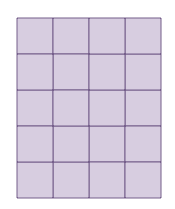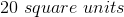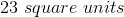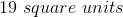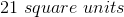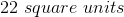There aresquares within the shape.